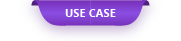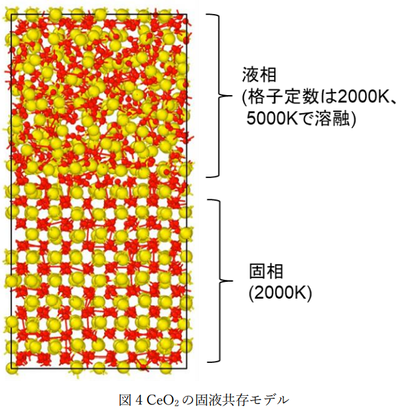The neural network molecular dynamics method (NNMD) enables faster simulations than first-principles calculations and more ...

# Analysis of thermal properties of CeO2 using neural network molecular dynamics

The neural network molecular dynamics method (NNMD) enables faster simulations than first-principles calculations and more accurate simulations than existing molecular dynamics calculations, but the first-principles for creating interatomic potentials for use in NNMD. The challenge is the need for large amounts of computationally-based teacher data. In this case, the potential learning is performed in a short time by simultaneously executing a large amount of first-principles calculations at once using a cloud system to create learning data, and CeO 2 is difficult to reproduce in the conventional force field . We will introduce an example of analyzing the behavior of the solid-liquid interface using NNMD.

### 1. Creation of training data for NNMD potential

Figure 1 shows the computational model used to create the training data for CeO 2 . In addition, Table 1 shows the details of the calculation conditions. Deep MD-kit  was used for potential machine learning.

### 2. Calculation result

① Specific heat and coefficient of thermal expansion

The specific heat and coefficient of thermal expansion of CeO 2 were calculated using the created potential function and molecular dynamics code LAMMPS . The calculation conditions are shown in Table 2. The specific heat and coefficient of thermal expansion were evaluated using the entropy and the temperature derivative of the volume. Figure 2 shows the calculation results of the temperature change and specific heat of the entropy. Figure 3 shows the calculation results of the volume temperature change and the coefficient of thermal expansion. It can be seen that the calculation results correspond well with the actual measurement .

② Melting point

In the calculation of the specific heat and the coefficient of thermal expansion in Fig. 2 and Fig. 3, the melting point could not be evaluated accurately, so the melting point was evaluated using the solid-liquid coexistence model. Table 3 and Figure 4 show the melting point calculation conditions and calculation model.

Figure 5 shows the calculation results of the temperature dependence of enthalpy and density. The enthalpy and density became discontinuous at 2650K to 2750K near the melting point of CeO2, and it was confirmed that the solid phase and the liquid phase coexisted near the melting point.

The MD animations at 2650K and 2700K near the melting point are shown on the left. It can be confirmed that the liquid phase is crystallized at 2650K, while the solid phase is melted at 2700K.

### 3. References

 Han Wang, Linfeng Zhang, Jiequn Han, and Weinan E. “DeePMD-kit: A deep learning package for many-body potential energy representation and molecular dynamics.” Computer Physics Communications 228 (2018): 178-184.

 N. Nelson, D Rittman, J. White et al., “An Evaluation of the Thermophysical Properties of Stoichiometric CeO2 in Comparison to UO2 and PuO2”, Journal of the American Ceramic Society, 97, 3652-3659 (2014)

Original Source from: https://ctc-mi-solution.com/ニューラルネットワーク分子動力学法を用いたceo2-2/